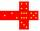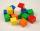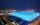# Cube + square root - math problems

#### Number of problems found: 22

• Cube surfce2volumeCalculate the volume of the cube if its surface is 150 cm2.
• Cuboid to cubeA cuboid with dimensions of 9 cm, 6 cm, and 4 cm has the same volume as a cube. Calculate the surface of this cube.
• The cubeThe cube has a surface area of 216 dm2. Calculate: a) the content of one wall, b) edge length, c) cube volume.
• Magnified cubeIf the lengths of the edges of the cube are extended by 5 cm, its volume will increase by 485 cm3. Determine the surface of both the original and the magnified cube.
• Wallpaper3750 cm square of wallpaper is needed to glue a cube-shaped box. Can Dad cut out the whole necessary piece of wallpaper as a whole if he has a roll of wallpaper 50 cm wide?
• Length of the edgeFind the length of the edge of a cube that has a cm2 surface and a volume in cm3 expressed by the same number.
• Cube surface and volumeThe surface of the cube is 500 cm2, how much cm3 will be its volume?
• Cube diagonalsCalculate the length of the side and the diagonals of the cube with a volume of 27 cm3.
• Area to volumeIf the surface area of a cube is 486, find its volume.
• Cube edgeDetermine the edges of the cube when the surface is equal to 37.5 cm square.
• The cubeThe surface of the cube is 150 square centimeters. Calculate: a- the content of its walls b - the length of its edges
• Cube - wallV kocke ABCDEFGH je ?. Aký je povrch kocky?
• Cube 6Surface area of one wall cube is 1600 cm square. How many liters of water can fit into the cube?
• Cube 6Volume of the cube is 216 cm3, calculate its surface area.
• Cube 8The surface of the cube is 0.54 m2. Calculate the length of the cube edge.
• Cube in a sphereThe cube is inscribed in a sphere with volume 7253 cm3. Determine the length of the edges of a cube.
• CubesOne cube is inscribed sphere and the other one described. Calculate difference of volumes of cubes, if the difference of surfaces in 257 mm2.
• HoleIn the center of the cube with edge 14 cm we will drill cylinder shape hole. Volume of the hole must be 27% of the cube. What drill diameter should be chosen?
• Cube wallSurface of first cube wall his 225 mm2. Second cube area is 60% of the surface of the first cube. Determine the length of the edge of the second cube (x).
• Cube diagonalDetermine the length of the cube diagonal with edge 37 mm.

Do you have an interesting mathematical word problem that you can't solve it? Submit a math problem, and we can try to solve it.

We will send a solution to your e-mail address. Solved examples are also published here. Please enter the e-mail correctly and check whether you don't have a full mailbox.

Please do not submit problems from current active competitions such as Mathematical Olympiad, correspondence seminars etc...